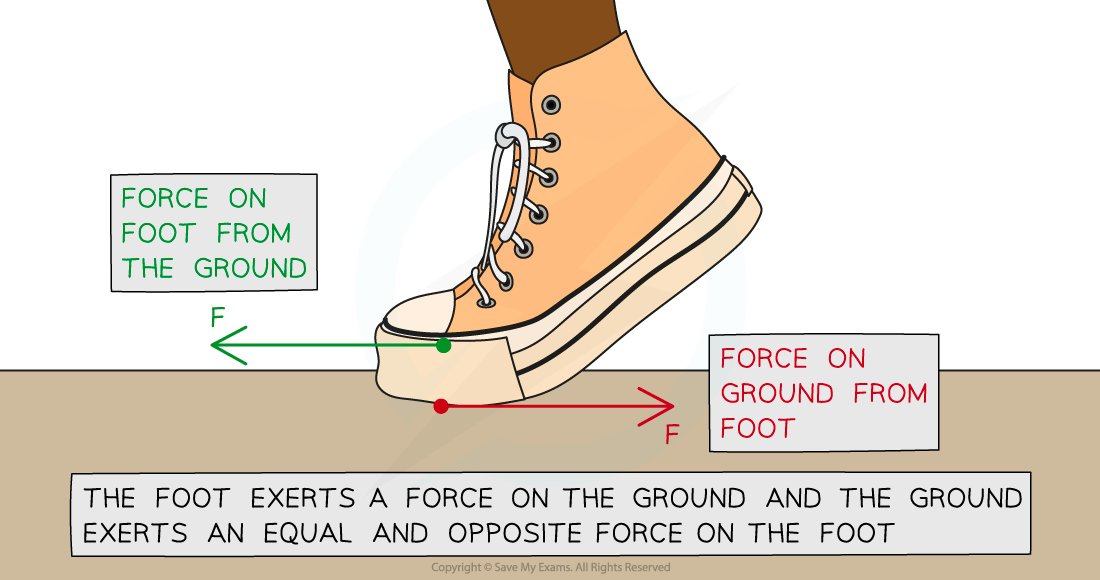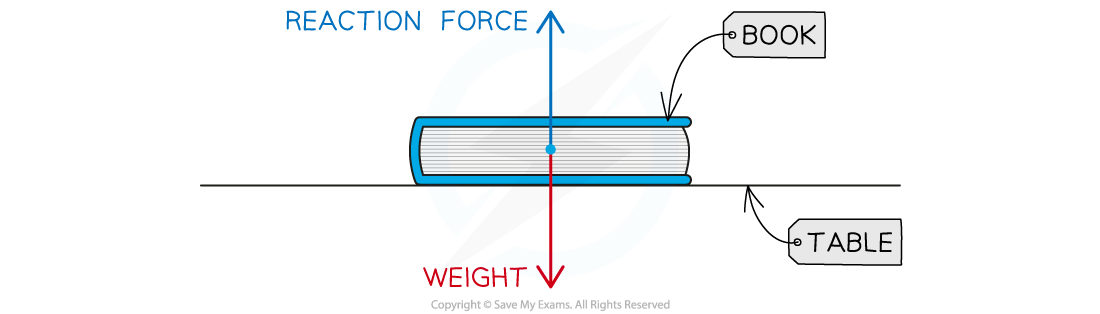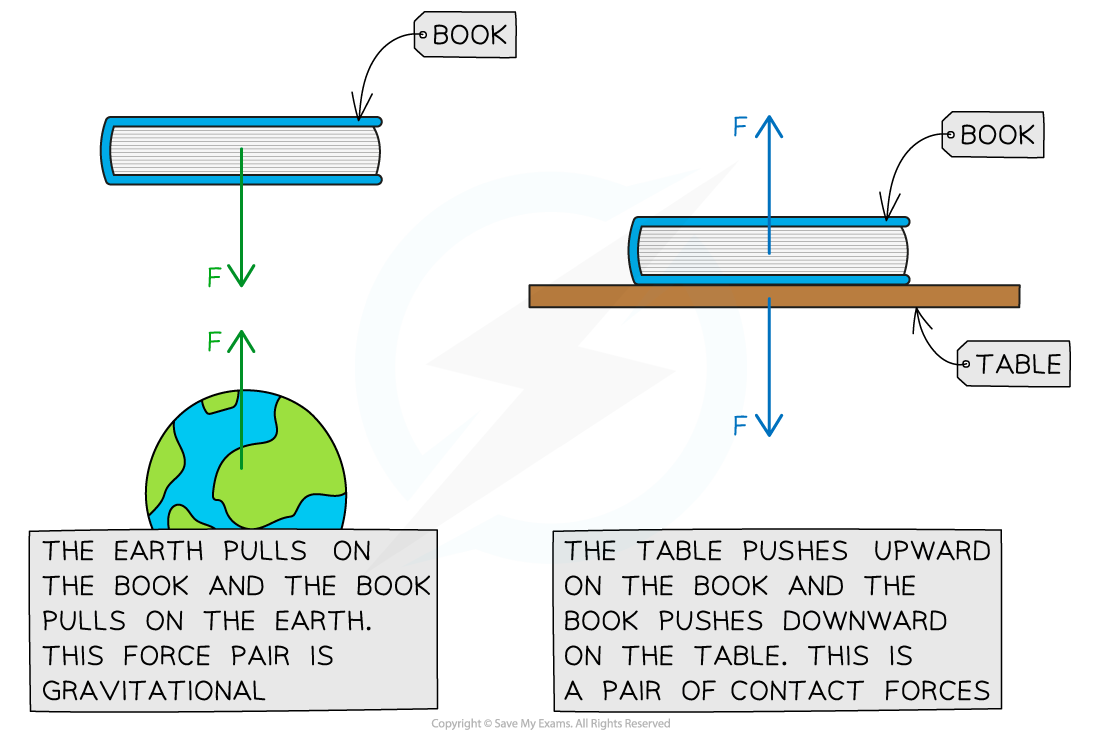# IB DP Physics: SL复习笔记2.2.4 Newton’s Third Law

### Newton’s Third Law

• Newton’s third law of motion states:

Whenever two bodies interact, the forces they exert on each other are equal and opposite

• Newton’s third law explains the following important principles about forces:
• All forces arise in pairs – if object A exerts a force on object B, then object B exerts an equal and opposite force on object A
• Force pairs are of the same type – for example, if object A exerts a gravitational force on object B, then object B exerts an equal and opposite gravitational force on object A
• Newton’s third law explains the forces that enable someone to walk
• The image below shows an example of a pair of equal and opposite forces acting on two objects (the ground and a foot):Newton's Third Law: The foot pushes the ground backwards, and the ground pushes the foot forwards

• One force is from the foot that pushes the ground backwards
• The other is an equal and opposite force from the ground that pushes the foot forwards

#### Worked Example

A physics textbook is at rest on a dining room table.Eugene draws a free body force diagram for the book and labels the forces acting on it.Eugene says the diagram is an example of Newton's third law of motion. William disagrees with Eugene and says the diagram is an example of Newton's first law of motion.By referring to the free-body force diagram, state and explain who is correct.

Step 1: State Newton's first law of motion

• Objects will remain at rest, or move with a constant velocity unless acted on by a resultant force

Step 2: State Newton's third law of motion

• Whenever two bodies interact, the forces they exert on each other are equal and opposite

Step 3: Check if the diagram satisfies the two conditions for identifying Newton's third law

• In each case, Newton's third law identifies pairs of equal and opposite forces, of the same type, acting on two different objects
• The diagram only involves one object
• Furthermore, the forces acting on the object are different types of force - one is a contact force (from the table) and the other is a gravitational force on the book (from the Earth) - its weight
• The image below shows how to apply Newton's third law correctly in this case, considering the pairs of forces acting:Step 4: Conclude which person is correct

• In this case, William is correct
• The free-body force diagram in the question is an example of Newton's first law
• The book is at rest because the two forces acting on it are balanced - i.e. there is no resultant force

#### Exam Tip

Remember that pairs of equal and opposite forces in Newton's third law act on two different objects. It's a really common mistake to confuse Newton's third law with Newton's first law, so applying this check will help you distinguish between them. Newton's first law involves forces acting on a single object.These differences are shown in Scenario 1 (Newton's first law) vs. Scenario 2 (Newton's third law)